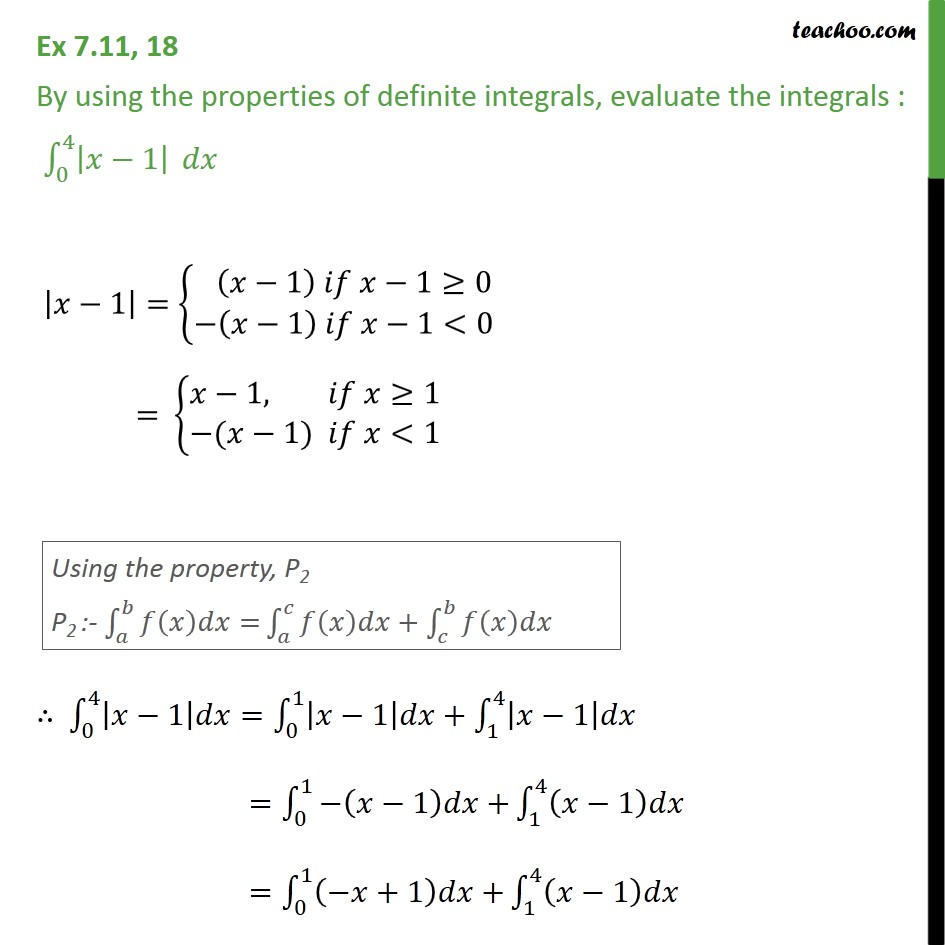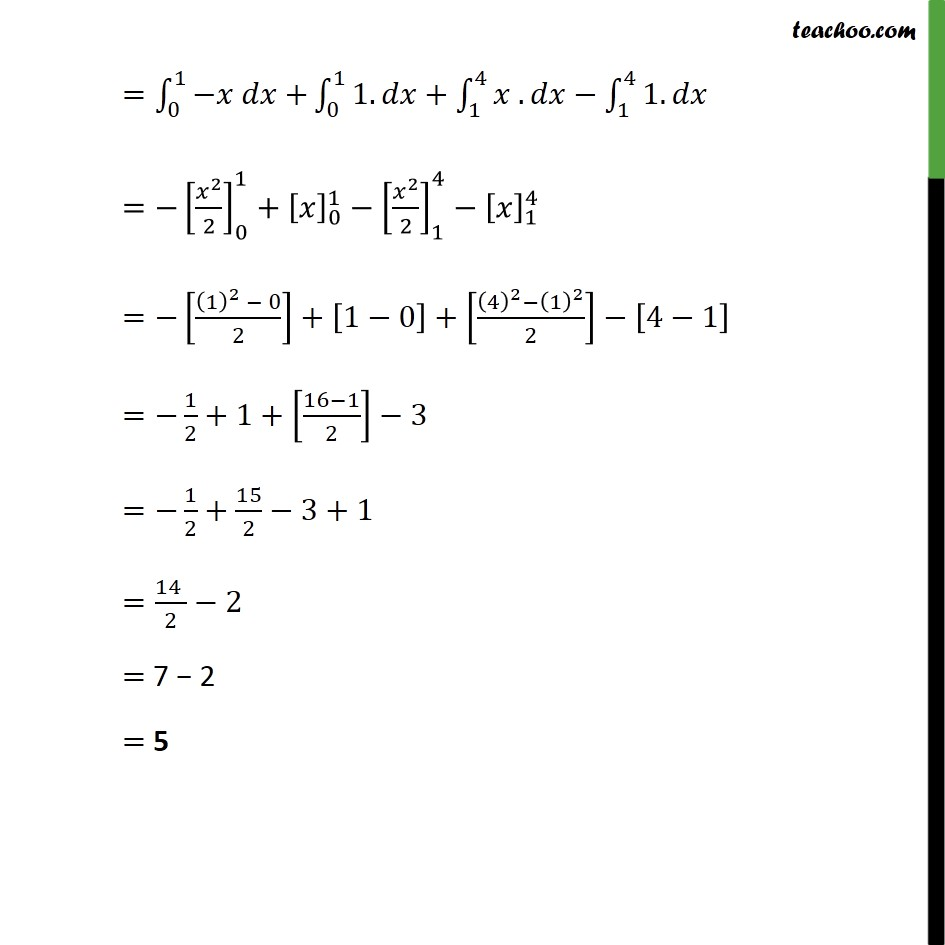1. Chapter 7 Class 12 Integrals
2. Concept wise
3. Definite Integration by properties - P2

Transcript

Ex 7.11, 18 By using the properties of definite integrals, evaluate the integrals : _0^4 | 1| = _0^1 + _0^1 1. + _1^4 . _1^4 1. = [ ^2/2]_0^1+[ ]_0^1 [ ^2/2]_1^4 [ ]_1^4 = [((1)^2 0)/2]+[1 0]+[((4)^2 (1)^2)/2] [4 1] = 1/2+1+[(16 1)/2] 3 = 1/2+15/2 3+1 =(14 )/2 2 = 7 2 = 5

Definite Integration by properties - P2